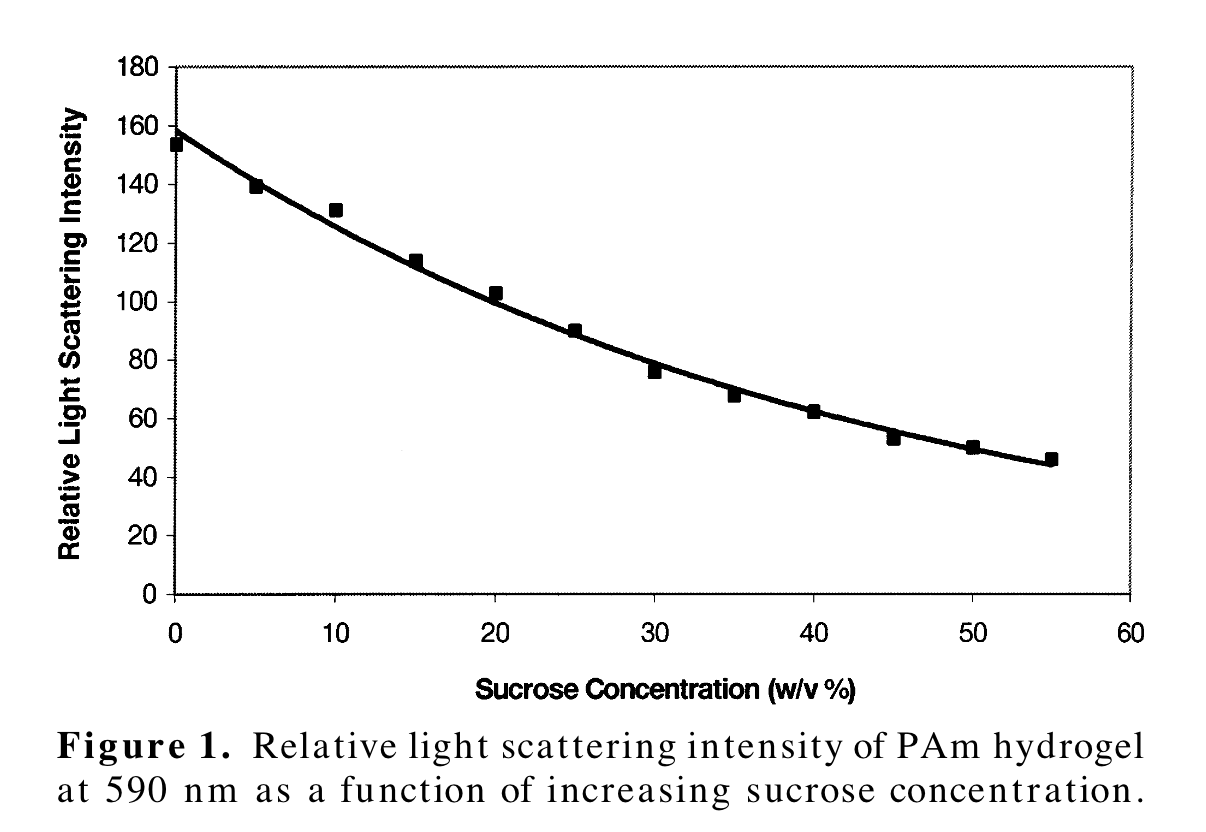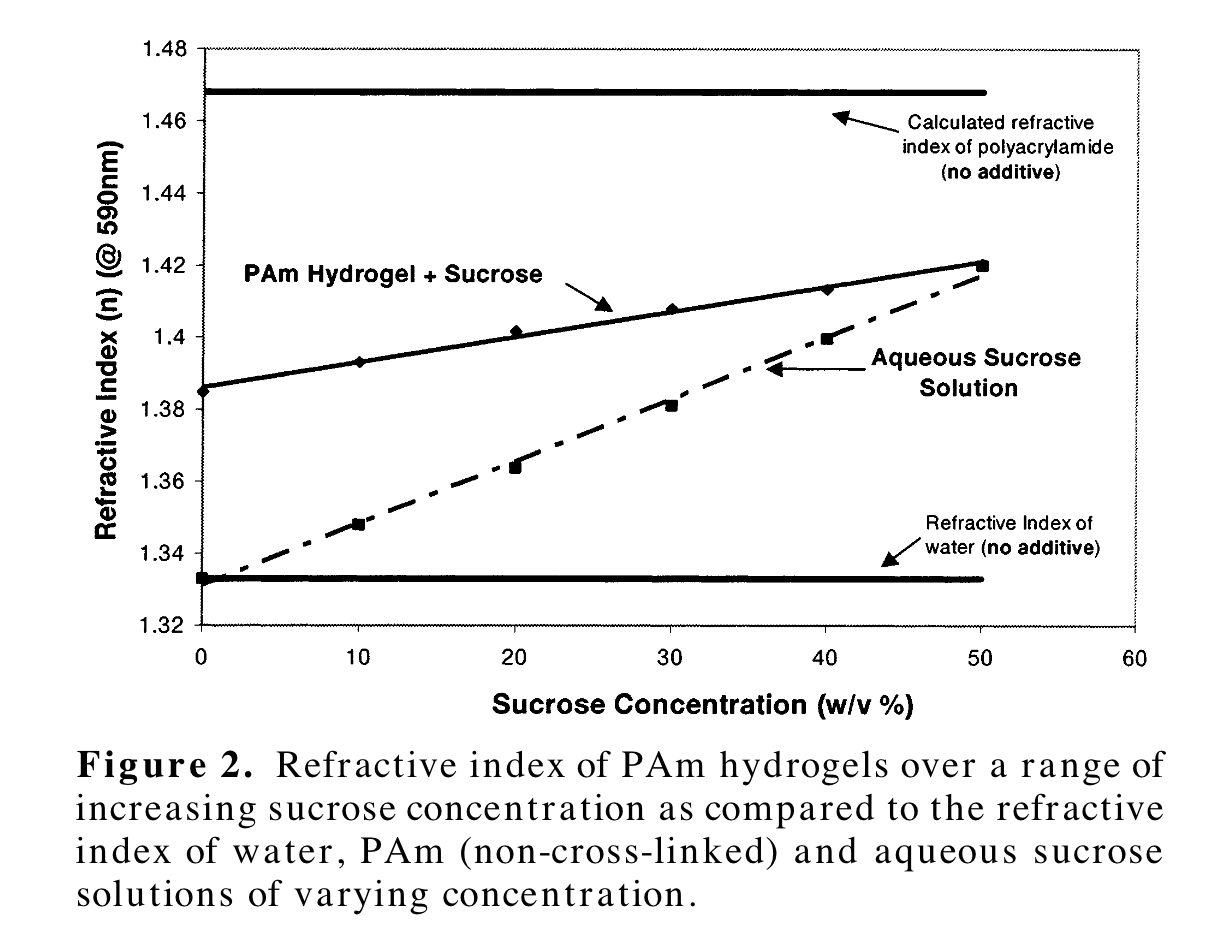# 202304011243

First published:

Last Edited:

Number of edits:

By increasing the refractive index of the surrounding aqueous media through the incorporation of additives, it may be possible to compensate for “refractive index inhomogeneities” formed within the developing hydrogel network. The observed result should be a reduction in the amount of light the hydrogel scatters.

The problem with the polyacrylamide gels is not only that they have a refractive index different from that of water (which can be solved by index-matching the surrounding medium), but that they scatter light. This can be described by the equation of the specific refraction:

$$\textrm{d}n/\textrm{d}c=(n_p-n_s)/c$$
where $n_p$ is the refractive index of the polymer network, $n_s$ is the refractive index of the solvent, and $c$ denotes the concentration of the polymer solution.

The approach in the paper is to add sucrose (because it does not interact chemically with a redox-type initiator like APS). By changing the refractive index of the solvent they effectively reduce the scattering of light from within the gel:On the other hand, adding sucrose increases the refractive index of the gel, like shown on the figure below:The question is how much sucrose can be added and still have a reliable protocol to immobilize nanoparticles.

These are the other notes that link to this one.